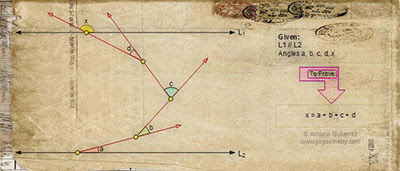## Monday, March 23, 2015

### Geometry Problem 550

Artwork of Geometry Problem 550.
Level: Mathematics Education, High School, Honors Geometry, College.

Click the diagram below to enlarge it.#### 1 comment:

1.Let A, B, C, D, X be the vertices containing angles a, b, c, d, x respectively.
Extend line BC to meet L1 at E and L2 at F.
Clearly <CEX = <BF L2 = a + b.
Extend CD to meet L1 at G.
Clearly <CGX = a + b + c.
Hence x = a + b + c + d